# Brachistochrone — are things really how you expect them to be?

“Art is a step from what is obvious and well-known toward what is arcane and concealed.”
- Khalil Gibran

One of the teachers in our university, who is teaching us Real Analysis 2 this semester, told us during one of his classes, “My this year’s resolution is, I would say ‘That’s obvious/trivial/clear’ less than last year.” And he also forbade us to say so in his classes. And I think that’s very important in a pure mathematics class. Because the things that seem obvious, are often very hard to prove rigorously; and your gut feeling or intuition doesn’t count as mathematical rigor.

Alright, backstory time over! Now let’s talk about a famous math (or physics?) problem. The problem was first proposed by mathematician Johann Bernoulli in the late 17th century. The problem is actually very easy to understand. So, the statement is: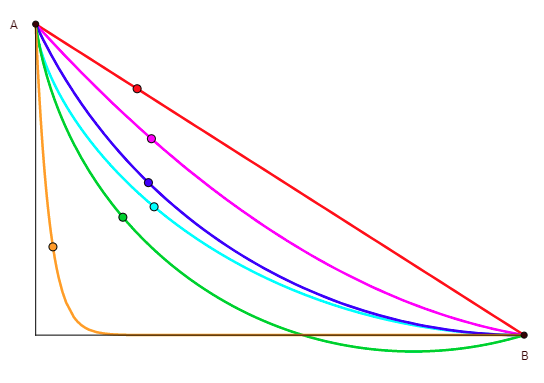There can be infinitely many paths from A to B. But which path allows us to travel in the shortest amount of time?

Suppose you have a point A and some other point B below but not directly below A. Now you have to find out the path from A to B, such that a particle can go from point A to point B in the shortest amount of time. The only acting force is gravity. And we live in an imaginary perfect world, so there is no friction, no air resistance.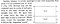The first shortest time and/or shortest path problem I encountered, from Perelman’s book.

I really like these shortest time and/or shortest path problems. The first time I saw one such shortest path problem (of course outside textbook) was from Yakov Perelman’s book Physics For Entertainment. (I attached the problem here) As a 6th grader who had literally zero knowledge about refraction of light or Snell’s law, let alone Fermat’s principle, the problem really amazed me. Because the obvious answer seems to be just the straight line that connects A and C. But the speed in Turf is more than the speed in Sand. So the messenger would want to travel more in Turf than sand. And the optimal solution looks like this: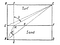Solution to the cavalry-messenger problem from Perelman’s book, AMC is the path that takes the least amount of time

If you know Snell’s law, you know that that’s how light travels when it goes from one medium from another (refraction of light). This really defied my intuition. :0

The next time I can recall of seeing another such shortest time and/or shortest path problem was in my 11th grade. Our physics textbook has a dedicated chapter on vector, and that chapter contains a problem of crossing river in shortest time. I was not as amazed to see that shortest distance doesn’t always give us the shortest time, because I learnt from past :)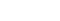The crossing river problem. There is a river, with distance d between the two banks, flowing to the right. If you row your boat at a specific angle θ, your resultant direction will be perpendicular and that’s the shortest distance. However, the shortest time can be achieved if you row perpendicularly.

Now, why people intuitively think that shortest distance should give us the shortest time? You’ve probably learnt something like this in elementary school or middle school:

Firstly, when you’re thinking about minimizing time, you probably think about minimizing distance. But you ignore the possibility that minimized distance can give us a lower speed, so we might not get the optimal time (this is the case in the crossing river problem; shortest distance reduces the vertical component of the boat’s velocity). And secondly, this formula works for constant speed. But most scenarios involve changeable velocity (or speed), so we can’t directly apply this middle school formula (when light goes from one medium to another, or the cavalryman goes from sand to turf, the speed changes; so using this middle school formula to find out the shortest time doesn’t make any sense).

Now let’s get back to the Bernoulli problem I started with. Even if you thought for a brief moment that the shortest time might be achieved with the shortest distance, you’re probably thinking otherwise now. If that’s the case, you’re in the right track. And it’s fairly intuitive to see why there should be better paths than the linear shortest path. Because if you make the path steeper, the component of gravitational force increases, so the velocity increases. But if the path is too long, this increase in velocity might not be helpful. So we need to find a balance between shortness and steepness of the curve.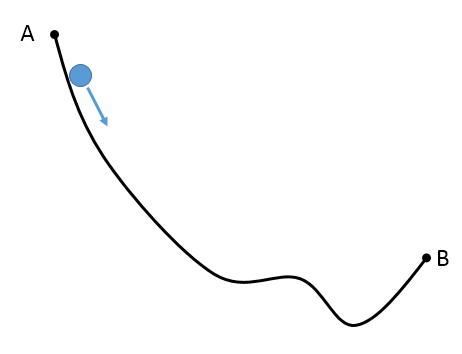This curve is steeper than the straight line, so it obtains a higher velocity. But the path is also longer. How can we balance shortness and steepness?

# So how did Bernoulli solve it?

The approach Bernoulli took, instead of thinking about the problem from dynamics’ perspective, he approached this problem from optics’ perspective. Instead of “a particle falling due to gravity”, he rephrased the problem as “a beam of light passing through different media (refraction of light)”. This is, indeed, a jaw-dropping idea. But the intuition becomes clear soon. Fermat proved that light always follows the path that takes the least time. And refraction of light can be explained by Fermat’s principle. So the idea of following light to get the path with least time did not really come out of nowhere.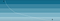If there were 5 media between A and B, this is the path light would take to reach in the shortest possible time

One quick thing to notice, the media should be in the descending order of “light density”. Because the velocity increases as the particle goes downwards, so it should be the same for the light. And the less the light density, the more the velocity.

Now Snell’s Law comes into play here. When light goes from one medium to another, if we draw a perpendicular line at the contact point, we get an angle θ between the path of light and the perpendicular line. Snell’s law tells us that, the ratio sin(θ)/v is always constant, where v denotes the speed of light in that medium.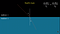Snell’s Law, sin(θ)/v always stays constant. Image Courtesy: 3blue1brown (I edited a bit)

Now Bernoulli notices another fact that gives him information about the velocity. He finds out that, the velocity is proportional to the square root of distance from the top.

That’s not very much hard to prove. It follows directly from the conservation of energy. The change in potential energy from point A to point P is mgy, where m is the mass of the particle. All energy are conserved, so this change in potential energy fully converts into kinetic energy. Initially kinetic energy was 0, so the kinetic energy at point P becomes mgy. Therefore,

Okay back to layer of various media. Here every media obeys Snell’s law, and velocity is proportional to the square root of vertical distance. In reality, velocity is instantaneously changing, so there are actually infinitely many lairs that are infinitesimally thin. So we can think of it as a limiting process. When the number of layers approaches infinity, the velocity becomes tangent to the curve. So now, the limiting Snell’s law becomes

So our desired curve has a very special property. That is: you take any point on the curve, you draw the tangent line at that point, and you draw a vertical line through that point; these two lines make an angle, the sine of the angle divided by the vertical distance stays constant always.

There is only one type of curve that satisfies this criteria, and Bernoulli recognized it immediately. This type of curve is called Cycloid. So we got our answer, the shortest time curve would be a cycloid connecting the starting point and the ending point.

# But what exactly is a cycloid? And why does it have this nice property?

Suppose you have a wheel rolling on a flat surface. You fix a point on the wheel. As you roll the wheel, you trace the locus of that point. The curve you get — that is called a cycloid.The red curve, traced from a rolling wheel, is a cycloid.

The definition of cycloid doesn’t really manifest why a cycloid should have our desired sin(θ)/√y is constant property. This can be proved using differential calculus, but I wanna present a nice visual proof given by mathematician Mark Levi.

Suppose P is any point on the cycloid (blue curve), and green circle is the wheel that generates this cycloid. X is the point of contact between the circle and the plane (red). Y is the diametrically opposite point (aka antipod) of X. Since the cycloid is created from a rolling circle, at any given time X is the instant center of rotation of the circle, so every point on the circle rotates around X at that moment and performs a circular motion around that point. So the yellow dotted circle represents the instantaneous circular motion of P, so it’s tangent to the cycloid. As it is tangent to the cycloid, the tangent line to the cycloid at P must also be tangent to the yellow dotted circle.

As ∠XPY is a right angle (because it’s on a semicircle), PY is tangent to the yellow circle, hence it’s tangent to the cycloid at P. Now, the angle θ between the tangent line and the vertical line is the pink angle pointed in the photo. Also, it can easily be shown that θ=∠PYX=∠PXZ.

where R is the radius of the green circle. Now, the green circle is rolling forwards and thus constructing the blue cycloid. The green circle never changes its shape, thus R is always constant. So we can arrive at a conclusion that sin(θ)/√y is indeed a constant.

Bernoulli’s truly amazing idea, along with Mark Levi’s surprisingly simple proof, makes one of the most beautiful solution to this problem. Here is a visual demonstration of this problem. The blue curve is the cycloid, and falling through this path takes the least amount of time.

This problem is popularly known as the Brachistochrone Problem. Brachistochrone is the Greek word for “shortest time”. Two of my favorite YouTube channels made two exceptionally good videos about Brachistochrone. Grant Sanderson from 3blue1brown collaborated with mathematician Steven Strocatz and they explained both Bernoulli’s and Mark Levi’s solutions. In fact, this article (so far) is mainly influenced by that 3b1b video.

Another video I can mention, is from Vsauce. In the video Michael Stevens and Adam Savage constructs a cycloid and demonstrates that cycloid is indeed the curve with fastest descent.

But, this article is not over yet. I wanna wrap this up with a solution I came up with. It’s not very elegant or beautiful as Bernoulli’s solution. But it is somewhat pleasing to me. (By the way, I googled afterwards, and found out that I’m not the only person who came up with this solution.)

In my solution, I wanna show that cycloid is the curve with fastest descent. Firstly, I reverse the direction of y-axis. This means, y-axis is positive in downwards direction. So g acts in the positive y-direction. Then I’ve divided my solution into several parts.

## What is the general equation of a cycloid?

Here, the blue curve is a cycloid. It is traced by the green circle. And P is a random point on the cycloid, and we want to calculate the coordinates of P. Notice that, AX is exactly the same as the arc PX. Because the point P was in the place of A at the beginning. As the green circle rolls, P travels arc PX distance. But the circle’s contact point with the red plane travels AX distance. These two must be equal, so

where R is the radius of the green circle, and θ=∠POX. We draw PQ⊥OX. Now PQ=R sinθ and OQ=R cosθ. So, the x-coordinate of P is AX-PQ=Rθ-R sinθ, y-coordinate of P is XQ=XO-OQ=R-R cosθ. Thus we get a parametric equation for cycloid, that is

where 0≤θ≤2π. So we got a general formula to describe cycloid.

## Alright, time for a shift in perspective.

Now we define a new coordinate system. We define a new d-φ coordinate system and convert our regular x-y coordinate system as follows

where 0≤φ≤2πd. Now, this may seem a little familiar to you. If we take d²=R and φ/d=θ, these are the exact same equations as the parametric equations for cycloid. What purposes does this new coordinate system serve? We assume that our initial startpoint A is the origin. If d stays constant, we get a cycloid-shape path (because d constant means R constant, and in the parametric equation for cycloid R is a constant that denotes the radius of the circle). For a non-cycloid-shape, we need d to be varying. So all I need to prove is, for the fastest descent d needs to be a constant for every point in the path from A to B.

Now a natural question arises. Why did I choose this d-φ coordinate system? Couldn’t I just use R-θ instead? Yes, I initially tried with R-θ, but the calculations got pretty messy after some point. Then I realized that a “magical substitution” can bring upon a drastic change. The magical substitution is this d-φ system.

If you’ve been reading things carefully, you might have a question in mind. How do we know that this coordinate system works? We haven’t proved that every point on the x-y plane can be written as d-φ in a unique way?

To prove it, we shall consider a function defined on (0,2π].

Then f(2π)=0. By L’Hopital rule

f is continuous on (0,2π]. So by intermediate value theorem, for every y/x≥0, there exists some θ such that y/x=f(θ). R=y/(1-cos θ) ensures us that we can find such R too. If we can find R-θ, we can find d-φ too. Thus for every point with positive x coordinate and non-negative y coordinate, we can always find such d-φ.

But it does not ensure that this d-φ coordinate will be unique. However, we can prove it with a fairly simple idea. If we can show that f ’(x)<0 for every x, then it follows that the θ for which y/x=f(θ) holds is unique (convince yourself why this is true). If we calculate the derivative,

which is obviously true when x≤2π. So f ’(x)<0 for every x, hence the d-φ coordinate is unique.

Now, we recall the equations for x-y and d-φ conversion again.

x and y are functions of d and φ. Applyling chain rule for differentiating by t, we get

whoo! I think I wrote them correctly. Pardon me if there is some typo. [differentiation with respect to time is written as a tiny dot above the variable, hope it makes sense]

Recall that, we proved earlier v²=2gy. And v is actually ds/dt , where ds is the tiny change in position. By Pythagorian Theorem, you can easily verify that ds=√(dx² + dy²). Because if we take smaller and smaller change in position, ds will look like a line, and then you can apply Pythagorian Theorem.

I skipped the middle steps, because they are just boring calculations, and writing them up is a lot of work.

Now we are almost done. Notice that, squares are always non-negative, so

This is true at all points, except A. Because A has y coordinate 0, so we can’t cancel out the y . Now, if we wanna find out the final φ, (suppose the final time is T)

That means, the least time is φ/g. And this time can be achieved when we have equality case to all the inequalities. Therefore, in the least time case

It’s easy to verify that the former case leads to contradiction. So d stays constant throughout the time. Hence, the path from A to B is a cycloid. Our proof is done.

If you’re reading this, I gotta praise your patience. I guess I’ll call it a day now. If you have any suggestions about new articles/improvements on this article/any questions, don’t hesitate to ask. You can comment here, or email me, or send me a message on instagram, or send me a message on twitter, or send me a message on discord (atonu_#1514). Adios!

“Mathematics consists in proving the most obvious thing in the least obvious way.”
— George Polya

I like to think I’m x% of a philosopher and y% of an artist, where x is larger than y.

## More from Atonu Roy Chowdhury

I like to think I’m x% of a philosopher and y% of an artist, where x is larger than y.